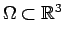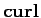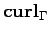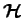Next: Bibliography

Project:

Computational Cohomology of Finite Element Meshes

 Researcher(s) : Prof. Dr. R. Hiptmair Funding : no external funding Duration : ongoing project

Description. Scalar potentials are a very convenient tool in computational electromagnetics when it comes to modelling irrotational vectorfields. For instance, ifdenotes a topologically trivial bounded domain, we have(1)

This amounts to the Poincaré lemma of the calculus of differential forms cast in the language of vector analysis. The statement (1) remains true, when replacingwith a simply connected surfaceandby the surface rotation.

There is a counterpart of (1) for co-chains on topologically simple simplicial complexes: if a 1-co-chain has zero rotation for all bounding cycles, it will the discrete gradient of 0-co-chain, that is, a function defined on the vertices of the complex. This is relevant for numerics, because co-chains are closely related to discrete differential forms, which provide popular finite element schemes for electromagnetic fields, for instance.

However, (1) and its discrete counterparts break down, whenand the simplicial complex have a non-vanishing first Betti number, which measures the number of handles of''. In this case, (1) has to be modified into(2)

whereis a fixed low dimensional co-homology space. In the discrete setting, that is, for co-chains,can be determined by

• finding a fundamental set of non-bounding edge cycles for the case of a simplicial mesh on a surface,
• finding a full set of abstract Seifert surfaces (cuts'')for the case of a 3D triangulation.
The first task can efficiently be solved using graph theoretic algorithms [HO02]. For the second fairly expensive matrix factorizations can do the job [GK01,RDB02]. Once the cuts are found,arising from taking the gradients of functions with jump 1 across the cut.

It is the goal of the project to investigate whether there are efficient algorithms for solving the discrete cut problem in three dimensions. By efficient we mean, that the computational effort should scale linearly in the size of the triangulation.

Activities. Currently, a term project by J. Kayatz is experimentally examining the use of edge contractions [DEGN99] for mesh simplifications.Next: Bibliography
Prof. Ralf Hiptmair 2004-11-04